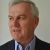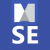# What is the best way to use formulas to calculate Time Value of Money (TVM)?

lonemccord shared this question ago

I suppose we can use the excel link to do this, but it would be great if we could have the formula built in. It would be super useful for budget predictions and other Financial planning topics. It is an elementary part of my business and I would love to use it in MindmanagerI've written a post on my blog outlining how to use MM formulas to undertake TVM calculations - and also more generally on how to use them with exponentiation: Logic, time and money: some MindManager formula surprises (Part 1) | Sociamind

## Replies (5)1

I'm certainly no expert at this but it is possible to write simple TVM calculations using MindManager formulas - much to my surprise, these actually allow exponential calculations.

The trick is incorporating time periods and intervals into these formulas, as MindManager does not make date numbering available in the same way Excel does. However, the MAP add-in does this indirectly, by making available extended topic properties such as Duration and Effort (both of these in minutes, hours, days, weeks and months), Days to Task Start, Days to Task Due and Total Days to Task Due.

The following is a simple example I set up based on the following formula (borrowed from Time Value of Money (TVM) Definition (investopedia.com)):

FV = PV x [ 1 + (i / n) ] (n x t)

- which assumes the following:

• FV = Future value of money
• PV = Present value of money
• i = interest rate
• n = number of compounding periods per year
• t = number of years

First, I used the following formula to calculate the number of years from the Duration Months property provided by MAP:

[t]=[Duration Months (#TDmths)]/13

Then I added the following formula:

[FV]=([PV]*((1+[i]/[n])^([n]*[t])))

The attached image shows the outcome based on a two-year period.1

Impressive, looks like I'm buying MAP!1

Strictly speaking if you stick to duration and effort only and can work with days then you don’t need MAP, but it’s useful as it handles the conversion into weeks and months. Also, if you want some of the other extended topic properties I mentioned like Days to Task Due then MAP is essential.

You might also find this thread on other undocumented logic operators in formulas useful: https://community.mindmanager.com/topic/127-please-retain-this-undocumented-but-very-useful-feature-in-formulas2

I've written a post on my blog outlining how to use MM formulas to undertake TVM calculations - and also more generally on how to use them with exponentiation: Logic, time and money: some MindManager formula surprises (Part 1) | Sociamind---